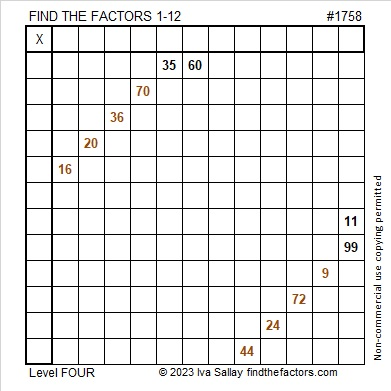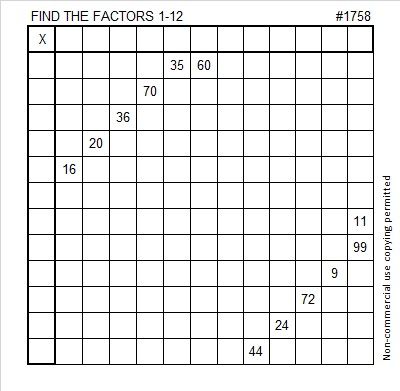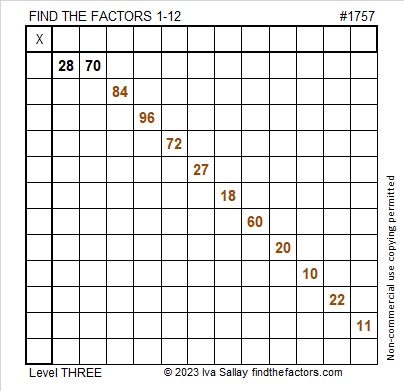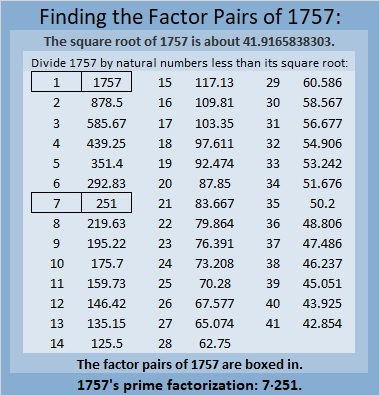# 1758 Two-Shillelagh O’Sullivan

### Today’s Puzzle:

When I was looking for the song about the shillelagh for my previous post, I found another one called Two-Shillelagh O’Sullivan also by Bing Crosby. It wasn’t a song from my childhood, but it inspired me to make a puzzle with two shillelaghs anyway. In the song, O’Sullivan wears these walking sticks in a holster and can draw them quicker than anyone can draw a gun. He was impossible to beat.

This two-shillelagh puzzle is also a bit difficult to beat. You’re not going to let that stop you from trying, are you? Just use logic and your knowledge of the multiplication table.

Write the numbers from 1 to 12 in the first column and again in the top row so that those numbers are the factors of the given clues.Here’s the same puzzle in black and white:### Factors of 1758:

• 1758 is a composite number.
• Prime factorization: 1758 = 2 × 3 × 293.
• 1758 has no exponents greater than 1 in its prime factorization, so √1758 cannot be simplified.
• The exponents in the prime factorization are 1, 1, and 1. Adding one to each exponent and multiplying we get (1 + 1)(1 + 1)(1 + 1) = 2 × 2 × 2 = 8. Therefore 1758 has exactly 8 factors.
• The factors of 1758 are outlined with their factor pair partners in the graphic below.### More About the Number 1758:

1758 is the hypotenuse of a Pythagorean triple:
408-1710-1758, which is 6 times (68-285-293).

1758 is palindrome 8E8 in base 14
because 8(14²) + 13(14) + 8(1) = 1758.

# 1757 A Shillelagh Puzzle

### Today’s Puzzle:

When I was in elementary school, one of my teachers had us listen to a variety of songs for Saint Patrick’s Day including Bing Crosby’s It’s the Same Old Shillelagh. It’s almost Saint Patrick’s Day, so here’s a puzzle that looks a little like a shillelagh, an Irish walking stick.

Since this is a level-3 puzzle, begin with the clues at the top of the puzzle and work your way down using each number 1 to 12 once in the first column and once in the top row. The numbers you write and the given clues need to form a multiplication table. There is only one solution.Here is the same puzzle in black and white:### Factors of 1757:

• 1757 is a composite number.
• Prime factorization: 1757 = 7 × 251.
• 1757 has no exponents greater than 1 in its prime factorization, so √1757 cannot be simplified.
• The exponents in the prime factorization are 1 and 1. Adding one to each exponent and multiplying we get (1 + 1)(1 + 1) = 2 × 2 = 4. Therefore 1757 has exactly 4 factors.
• The factors of 1757 are outlined with their factor pair partners in the graphic below.### More About the Number 1757:

1757 is the difference of two squares in two different ways:
879² – 878² = 1757, and
129² – 122² = 1757.

1757 is palindrome 616 in base17
because 6(17²) + 1(17) + 6(1) = 1757.

# 1607 Shillelagh

### Today’s Puzzle:

A Shillelagh is an Irish wooden walking stick. This Shillelagh is keeping with our Saint Patrick’s Day theme, but it is a Find the Factors 1 to 14 puzzle.  Brutal! It will be a whole lot less tricky for you to solve because I made it a level 3 puzzle: The logic needed to solve the puzzle is built in. Just start with the clue at the top of the puzzle and work your way down cell by cell until you have found all the factors. So crack on!Print the puzzles or type the solution in this excel file: 14 Factors 1604-1612.

### Factors of 1607:

• 1607 is a prime number.
• Prime factorization: 1607 is prime.
• 1607 has no exponents greater than 1 in its prime factorization, so √1607 cannot be simplified.
• The exponent in the prime factorization is 1. Adding one to that exponent we get (1 + 1) = 2. Therefore 1607 has exactly 2 factors.
• The factors of 1607 are outlined with their factor pair partners in the graphic below.How do we know that 1607 is a prime number? If 1607 were not a prime number, then it would be divisible by at least one prime number less than or equal to √1607. Since 1607 cannot be divided evenly by 2, 3, 5, 7, 11, 13, 17, 19, 23, 29, 31, or 37, we know that 1607 is a prime number.

### More about the Number 1607:

1607 is the sum of two consecutive numbers:
803 + 804 = 1607.

1607 is also the difference of two consecutive numbers:
804² – 803² = 1607.

Did you notice what happened there? Try this next one:

1607² = 2582449.

1607²/2 = 1291224.5.

(1607-1291224-1291225) is a primitive Pythagorean triple.

Cool, isn’t it?